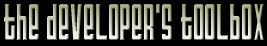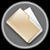This section of the archives stores flipcode's complete Developer Toolbox collection, featuring a variety of mini-articles and source code contributions from our readers.String Matching In Linear-Time   Submitted byHi, I noticed the queue seemed to be empty so here's a string matching implementation that runs in linear time, O(n+m) to be exact, where n is the length of the text to be searched and m is the length of the text to search for. It's based on the Knuth-Morris-Pratt algorithm (or my understanding of it at least :)). Although not an explicit game-programming issue, I hope this can be helpful for some people.

The single file actually compiles into a program that searches a file for a string. However here's an example:
 ```// ex1 string_search my_search; int pos = my_search.find_first("badfbhfbsdcbaca", "ba"); // ex2 std::vector

The first example will return 0, and the other 0 and ... eh, something else. You may use it as you like, but if you're going to give credits, give them where they are due.

/Andreas Magnusson
http://www.mdstud.chalmers.se/~md7amag/

 ```/* String search based on the Knuth-Morris-Pratt algorithm for linear running-time, O(m+n) where m=length of pattern and n=length of text. Written by Andreas Magnusson in November 2001 You may do as you please with this code, but don't blame me if anything goes wrong... */ #include #include #include #include typedef std::vector int_vec;class string_search { int_vec shifts; void compute_shifts(const std::string &pattern); public: int find_first(const std::string &text, const std::string &pattern); int_vec find_all(const std::string &text, const std::string &pattern);};// create the shift-lookup-table void string_search::compute_shifts(const std::string &pattern) { int next_shift = 0; shifts.clear(); shifts.push_back(0); // shift to the first character // start with the second character, since the shift to the first is always 0 for(int i = 1; i < pattern.length(); i++) { while(next_shift > 0 && pattern[next_shift] != pattern[i]) next_shift = shifts[next_shift]; if(pattern[next_shift] == pattern[i]) next_shift++; shifts.push_back(next_shift); } }// search the string and return when the first occurrence is found int string_search::find_first(const std::string &text, const std::string &pattern) { int next_shift = 0; compute_shifts(pattern); for(int i = 0; i < text.length(); i++) { while(next_shift > 0 && pattern[next_shift] != text[i]) next_shift = shifts[next_shift - 1]; if(pattern[next_shift] == text[i]) next_shift++; if(next_shift == pattern.length()) return i - (pattern.length() - 1); // found the first so return } return -1; }// search the string and put every occurence in a vector int_vec string_search::find_all(const std::string &text, const std::string &pattern) { int next_shift = 0; int_vec positions; compute_shifts(pattern); for(int i = 0; i < text.length(); i++) { while(next_shift > 0 && pattern[next_shift] != text[i]) next_shift = shifts[next_shift - 1]; if(pattern[next_shift] == text[i]) next_shift++; if(next_shift == pattern.length()) { positions.push_back(i - (pattern.length() - 1)); // found one, put in list next_shift = shifts[next_shift - 1]; // restart pattern with last shift } } return positions; }int main(int argc, char **argv) { if(argc <= 2){ cout << "Usage: " << argv << " filename searchpattern" << endl; return 0; } std::string pattern = argv; // read the file. Since the file is read like this all white-characters // are eaten, so a search including white-characters will fail... fstream fs; std::string text, temp; fs.open(argv, ios::in); while(!fs.eof()){ fs >> temp; text += temp; } fs.close(); // search the file string_search search; int_vec pos_list = search.find_all(text, pattern); // print out result std::vector::iterator it; cout << "Found " << pos_list.size() << " occurrences" << endl; for(it = pos_list.begin(); it != pos_list.end(); it++){ temp = text.substr(*it, pattern.length()); cout << "Pos=" << *it << " == " << temp << endl; } } ```